Anúncio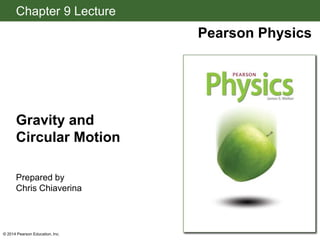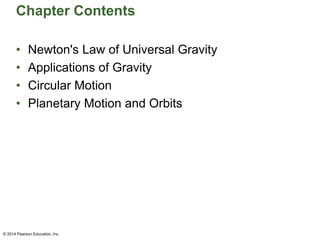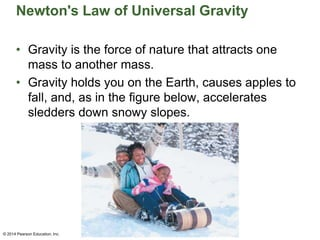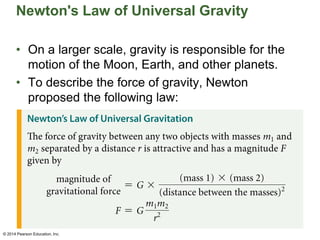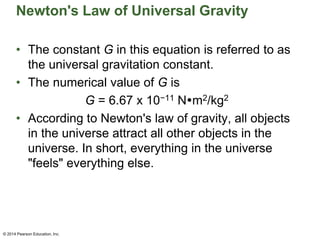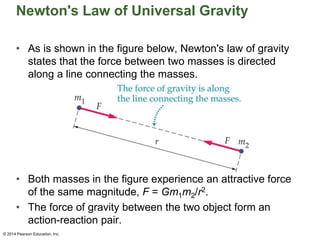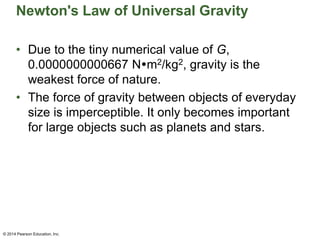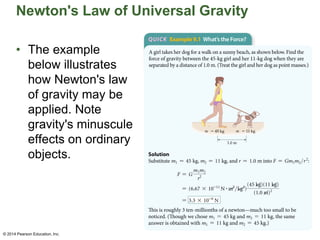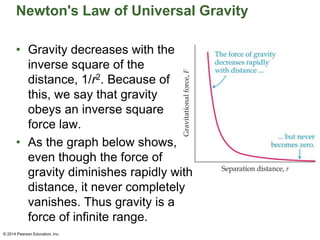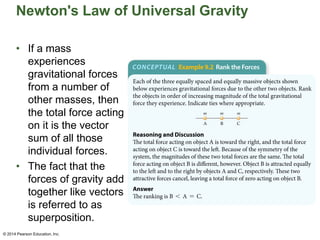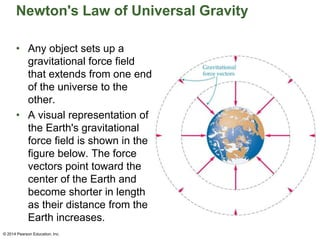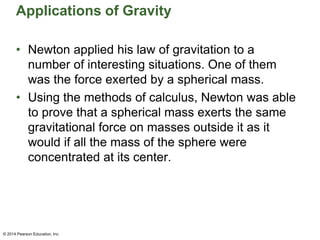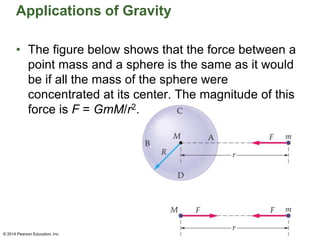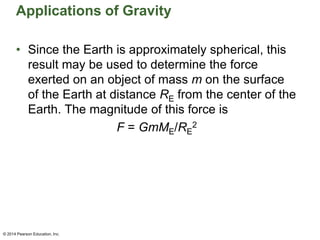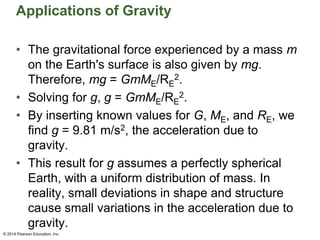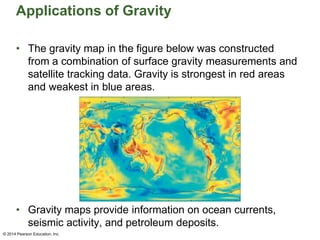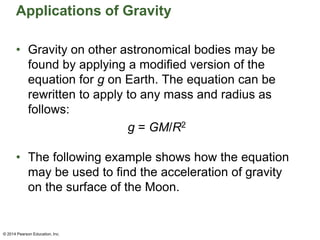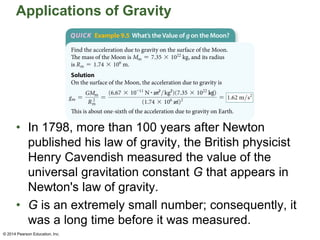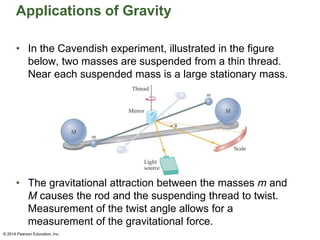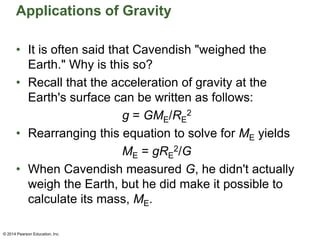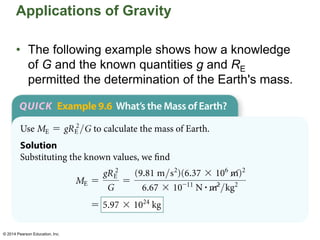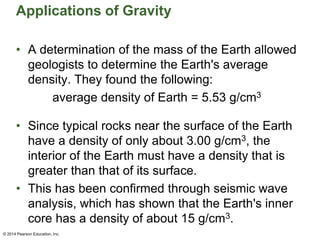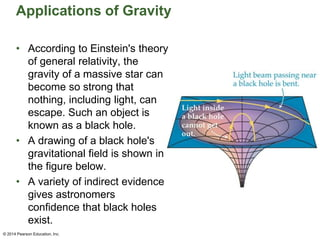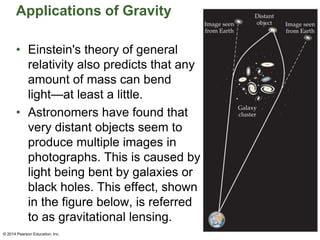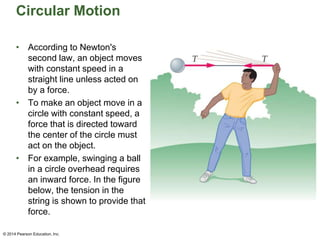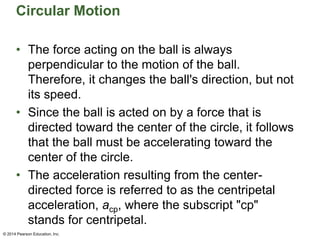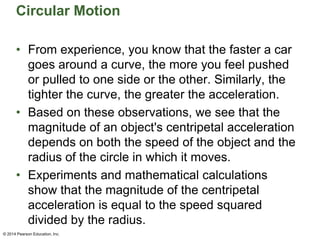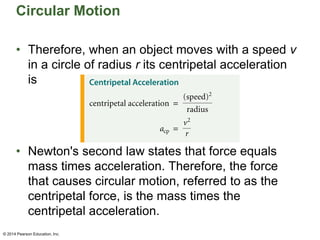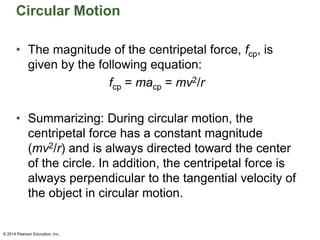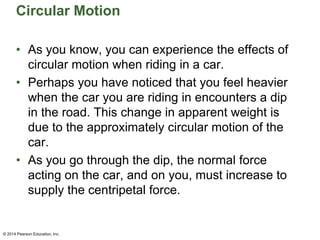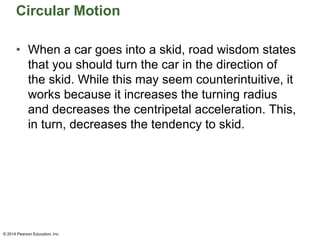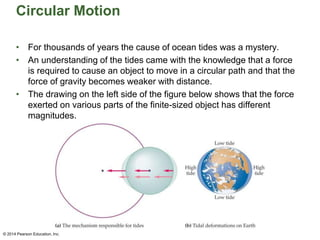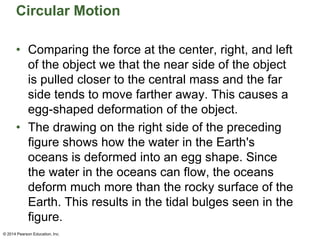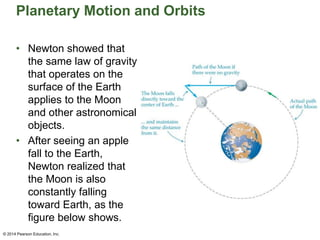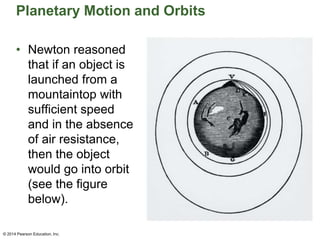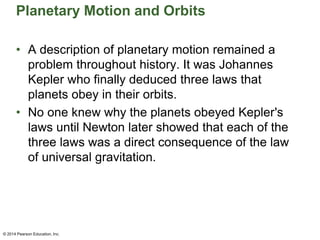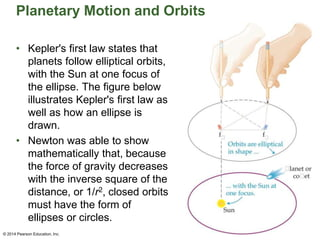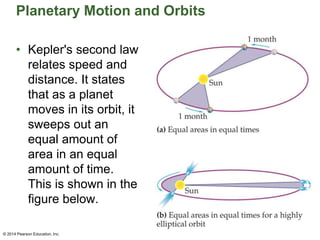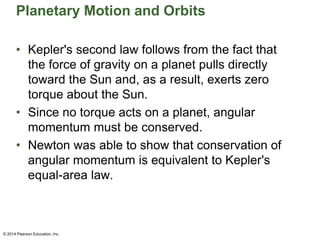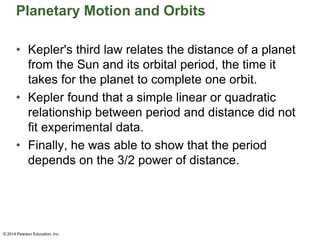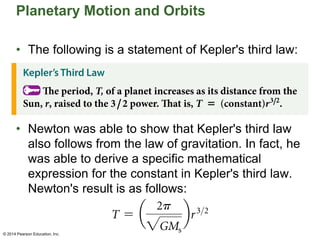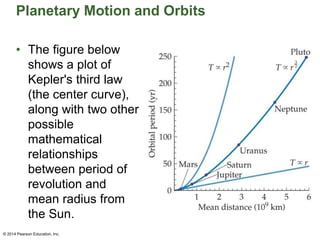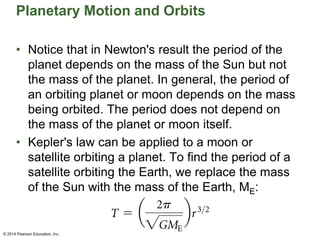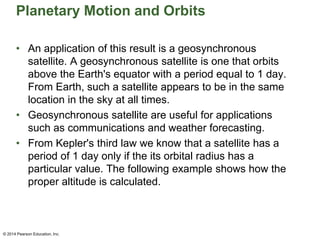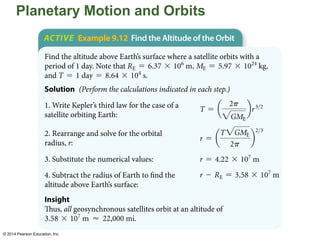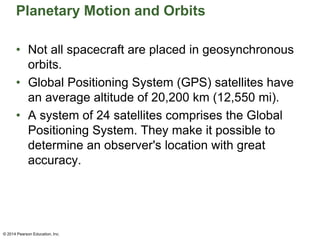1 de 46
Anúncio

### ch_09_PPT_lecture.pptx

1. Chapter 9 Lecture Pearson Physics Gravity and Circular Motion Prepared by Chris Chiaverina © 2014 Pearson Education, Inc.
2. Chapter Contents • Newton's Law of Universal Gravity • Applications of Gravity • Circular Motion • Planetary Motion and Orbits © 2014 Pearson Education, Inc.
3. Newton's Law of Universal Gravity • Gravity is the force of nature that attracts one mass to another mass. • Gravity holds you on the Earth, causes apples to fall, and, as in the figure below, accelerates sledders down snowy slopes. © 2014 Pearson Education, Inc.
4. Newton's Law of Universal Gravity • On a larger scale, gravity is responsible for the motion of the Moon, Earth, and other planets. • To describe the force of gravity, Newton proposed the following law: © 2014 Pearson Education, Inc. © 2014 Pearson Education, Inc.
5. Newton's Law of Universal Gravity • The constant G in this equation is referred to as the universal gravitation constant. • The numerical value of G is G = 6.67 x 10−11 Nm2/kg2 • According to Newton's law of gravity, all objects in the universe attract all other objects in the universe. In short, everything in the universe "feels" everything else. © 2014 Pearson Education, Inc.
6. Newton's Law of Universal Gravity • As is shown in the figure below, Newton's law of gravity states that the force between two masses is directed along a line connecting the masses. • Both masses in the figure experience an attractive force of the same magnitude, F = Gm1m2/r2. • The force of gravity between the two object form an action-reaction pair. © 2014 Pearson Education, Inc.
7. Newton's Law of Universal Gravity • Due to the tiny numerical value of G, 0.0000000000667 Nm2/kg2, gravity is the weakest force of nature. • The force of gravity between objects of everyday size is imperceptible. It only becomes important for large objects such as planets and stars. © 2014 Pearson Education, Inc.
8. Newton's Law of Universal Gravity • The example below illustrates how Newton's law of gravity may be applied. Note gravity's minuscule effects on ordinary objects. © 2014 Pearson Education, Inc. © 2014 Pearson Education, Inc.
9. Newton's Law of Universal Gravity • Gravity decreases with the inverse square of the distance, 1/r2. Because of this, we say that gravity obeys an inverse square force law. • As the graph below shows, even though the force of gravity diminishes rapidly with distance, it never completely vanishes. Thus gravity is a force of infinite range. © 2014 Pearson Education, Inc.
10. Newton's Law of Universal Gravity • If a mass experiences gravitational forces from a number of other masses, then the total force acting on it is the vector sum of all those individual forces. • The fact that the forces of gravity add together like vectors is referred to as superposition. © 2014 Pearson Education, Inc. © 2014 Pearson Education, Inc.
11. Newton's Law of Universal Gravity • Any object sets up a gravitational force field that extends from one end of the universe to the other. • A visual representation of the Earth's gravitational force field is shown in the figure below. The force vectors point toward the center of the Earth and become shorter in length as their distance from the Earth increases. © 2014 Pearson Education, Inc.
12. Applications of Gravity • Newton applied his law of gravitation to a number of interesting situations. One of them was the force exerted by a spherical mass. • Using the methods of calculus, Newton was able to prove that a spherical mass exerts the same gravitational force on masses outside it as it would if all the mass of the sphere were concentrated at its center. © 2014 Pearson Education, Inc.
13. Applications of Gravity • The figure below shows that the force between a point mass and a sphere is the same as it would be if all the mass of the sphere were concentrated at its center. The magnitude of this force is F = GmM/r2. © 2014 Pearson Education, Inc.
14. Applications of Gravity • Since the Earth is approximately spherical, this result may be used to determine the force exerted on an object of mass m on the surface of the Earth at distance RE from the center of the Earth. The magnitude of this force is F = GmME/RE 2 © 2014 Pearson Education, Inc.
15. Applications of Gravity • The gravitational force experienced by a mass m on the Earth's surface is also given by mg. Therefore, mg = GmME/RE 2. • Solving for g, g = GmME/RE 2. • By inserting known values for G, ME, and RE, we find g = 9.81 m/s2, the acceleration due to gravity. • This result for g assumes a perfectly spherical Earth, with a uniform distribution of mass. In reality, small deviations in shape and structure cause small variations in the acceleration due to gravity. © 2014 Pearson Education, Inc.
16. Applications of Gravity • The gravity map in the figure below was constructed from a combination of surface gravity measurements and satellite tracking data. Gravity is strongest in red areas and weakest in blue areas. • Gravity maps provide information on ocean currents, seismic activity, and petroleum deposits. © 2014 Pearson Education, Inc.
17. Applications of Gravity • Gravity on other astronomical bodies may be found by applying a modified version of the equation for g on Earth. The equation can be rewritten to apply to any mass and radius as follows: g = GM/R2 • The following example shows how the equation may be used to find the acceleration of gravity on the surface of the Moon. © 2014 Pearson Education, Inc.
18. Applications of Gravity • In 1798, more than 100 years after Newton published his law of gravity, the British physicist Henry Cavendish measured the value of the universal gravitation constant G that appears in Newton's law of gravity. • G is an extremely small number; consequently, it was a long time before it was measured. © 2014 Pearson Education, Inc. © 2014 Pearson Education, Inc.
19. Applications of Gravity • In the Cavendish experiment, illustrated in the figure below, two masses are suspended from a thin thread. Near each suspended mass is a large stationary mass. • The gravitational attraction between the masses m and M causes the rod and the suspending thread to twist. Measurement of the twist angle allows for a measurement of the gravitational force. © 2014 Pearson Education, Inc.
20. Applications of Gravity • It is often said that Cavendish "weighed the Earth." Why is this so? • Recall that the acceleration of gravity at the Earth's surface can be written as follows: g = GME/RE 2 • Rearranging this equation to solve for ME yields ME = gRE 2/G • When Cavendish measured G, he didn't actually weigh the Earth, but he did make it possible to calculate its mass, ME. © 2014 Pearson Education, Inc.
21. Applications of Gravity • The following example shows how a knowledge of G and the known quantities g and RE permitted the determination of the Earth's mass. © 2014 Pearson Education, Inc. © 2014 Pearson Education, Inc.
22. Applications of Gravity • A determination of the mass of the Earth allowed geologists to determine the Earth's average density. They found the following: average density of Earth = 5.53 g/cm3 • Since typical rocks near the surface of the Earth have a density of only about 3.00 g/cm3, the interior of the Earth must have a density that is greater than that of its surface. • This has been confirmed through seismic wave analysis, which has shown that the Earth's inner core has a density of about 15 g/cm3. © 2014 Pearson Education, Inc.
23. Applications of Gravity • According to Einstein's theory of general relativity, the gravity of a massive star can become so strong that nothing, including light, can escape. Such an object is known as a black hole. • A drawing of a black hole's gravitational field is shown in the figure below. • A variety of indirect evidence gives astronomers confidence that black holes exist. © 2014 Pearson Education, Inc.
24. Applications of Gravity • Einstein's theory of general relativity also predicts that any amount of mass can bend light—at least a little. • Astronomers have found that very distant objects seem to produce multiple images in photographs. This is caused by light being bent by galaxies or black holes. This effect, shown in the figure below, is referred to as gravitational lensing. © 2014 Pearson Education, Inc.
25. Circular Motion • According to Newton's second law, an object moves with constant speed in a straight line unless acted on by a force. • To make an object move in a circle with constant speed, a force that is directed toward the center of the circle must act on the object. • For example, swinging a ball in a circle overhead requires an inward force. In the figure below, the tension in the string is shown to provide that force. © 2014 Pearson Education, Inc.
26. Circular Motion • The force acting on the ball is always perpendicular to the motion of the ball. Therefore, it changes the ball's direction, but not its speed. • Since the ball is acted on by a force that is directed toward the center of the circle, it follows that the ball must be accelerating toward the center of the circle. • The acceleration resulting from the center- directed force is referred to as the centripetal acceleration, acp, where the subscript "cp" stands for centripetal. © 2014 Pearson Education, Inc.
27. Circular Motion • From experience, you know that the faster a car goes around a curve, the more you feel pushed or pulled to one side or the other. Similarly, the tighter the curve, the greater the acceleration. • Based on these observations, we see that the magnitude of an object's centripetal acceleration depends on both the speed of the object and the radius of the circle in which it moves. • Experiments and mathematical calculations show that the magnitude of the centripetal acceleration is equal to the speed squared divided by the radius. © 2014 Pearson Education, Inc.
28. Circular Motion • Therefore, when an object moves with a speed v in a circle of radius r its centripetal acceleration is • Newton's second law states that force equals mass times acceleration. Therefore, the force that causes circular motion, referred to as the centripetal force, is the mass times the centripetal acceleration. © 2014 Pearson Education, Inc. © 2014 Pearson Education, Inc.
29. Circular Motion • The magnitude of the centripetal force, fcp, is given by the following equation: fcp = macp = mv2/r • Summarizing: During circular motion, the centripetal force has a constant magnitude (mv2/r) and is always directed toward the center of the circle. In addition, the centripetal force is always perpendicular to the tangential velocity of the object in circular motion. © 2014 Pearson Education, Inc.
30. Circular Motion • As you know, you can experience the effects of circular motion when riding in a car. • Perhaps you have noticed that you feel heavier when the car you are riding in encounters a dip in the road. This change in apparent weight is due to the approximately circular motion of the car. • As you go through the dip, the normal force acting on the car, and on you, must increase to supply the centripetal force. © 2014 Pearson Education, Inc.
31. Circular Motion • When a car goes into a skid, road wisdom states that you should turn the car in the direction of the skid. While this may seem counterintuitive, it works because it increases the turning radius and decreases the centripetal acceleration. This, in turn, decreases the tendency to skid. © 2014 Pearson Education, Inc.
32. Circular Motion • For thousands of years the cause of ocean tides was a mystery. • An understanding of the tides came with the knowledge that a force is required to cause an object to move in a circular path and that the force of gravity becomes weaker with distance. • The drawing on the left side of the figure below shows that the force exerted on various parts of the finite-sized object has different magnitudes. © 2014 Pearson Education, Inc.
33. Circular Motion • Comparing the force at the center, right, and left of the object we that the near side of the object is pulled closer to the central mass and the far side tends to move farther away. This causes a egg-shaped deformation of the object. • The drawing on the right side of the preceding figure shows how the water in the Earth's oceans is deformed into an egg shape. Since the water in the oceans can flow, the oceans deform much more than the rocky surface of the Earth. This results in the tidal bulges seen in the figure. © 2014 Pearson Education, Inc.
34. Planetary Motion and Orbits • Newton showed that the same law of gravity that operates on the surface of the Earth applies to the Moon and other astronomical objects. • After seeing an apple fall to the Earth, Newton realized that the Moon is also constantly falling toward Earth, as the figure below shows. © 2014 Pearson Education, Inc.
35. Planetary Motion and Orbits • Newton reasoned that if an object is launched from a mountaintop with sufficient speed and in the absence of air resistance, then the object would go into orbit (see the figure below). © 2014 Pearson Education, Inc.
36. Planetary Motion and Orbits • A description of planetary motion remained a problem throughout history. It was Johannes Kepler who finally deduced three laws that planets obey in their orbits. • No one knew why the planets obeyed Kepler's laws until Newton later showed that each of the three laws was a direct consequence of the law of universal gravitation. © 2014 Pearson Education, Inc.
37. Planetary Motion and Orbits • Kepler's first law states that planets follow elliptical orbits, with the Sun at one focus of the ellipse. The figure below illustrates Kepler's first law as well as how an ellipse is drawn. • Newton was able to show mathematically that, because the force of gravity decreases with the inverse square of the distance, or 1/r2, closed orbits must have the form of ellipses or circles. © 2014 Pearson Education, Inc.
38. Planetary Motion and Orbits • Kepler's second law relates speed and distance. It states that as a planet moves in its orbit, it sweeps out an equal amount of area in an equal amount of time. This is shown in the figure below. © 2014 Pearson Education, Inc.
39. Planetary Motion and Orbits • Kepler's second law follows from the fact that the force of gravity on a planet pulls directly toward the Sun and, as a result, exerts zero torque about the Sun. • Since no torque acts on a planet, angular momentum must be conserved. • Newton was able to show that conservation of angular momentum is equivalent to Kepler's equal-area law. © 2014 Pearson Education, Inc.
40. Planetary Motion and Orbits • Kepler's third law relates the distance of a planet from the Sun and its orbital period, the time it takes for the planet to complete one orbit. • Kepler found that a simple linear or quadratic relationship between period and distance did not fit experimental data. • Finally, he was able to show that the period depends on the 3/2 power of distance. © 2014 Pearson Education, Inc.
41. Planetary Motion and Orbits • The following is a statement of Kepler's third law: • Newton was able to show that Kepler's third law also follows from the law of gravitation. In fact, he was able to derive a specific mathematical expression for the constant in Kepler's third law. Newton's result is as follows: © 2014 Pearson Education, Inc. © 2014 Pearson Education, Inc.
42. Planetary Motion and Orbits • The figure below shows a plot of Kepler's third law (the center curve), along with two other possible mathematical relationships between period of revolution and mean radius from the Sun. © 2014 Pearson Education, Inc.
43. Planetary Motion and Orbits • Notice that in Newton's result the period of the planet depends on the mass of the Sun but not the mass of the planet. In general, the period of an orbiting planet or moon depends on the mass being orbited. The period does not depend on the mass of the planet or moon itself. • Kepler's law can be applied to a moon or satellite orbiting a planet. To find the period of a satellite orbiting the Earth, we replace the mass of the Sun with the mass of the Earth, ME: © 2014 Pearson Education, Inc.
44. Planetary Motion and Orbits • An application of this result is a geosynchronous satellite. A geosynchronous satellite is one that orbits above the Earth's equator with a period equal to 1 day. From Earth, such a satellite appears to be in the same location in the sky at all times. • Geosynchronous satellite are useful for applications such as communications and weather forecasting. • From Kepler's third law we know that a satellite has a period of 1 day only if the its orbital radius has a particular value. The following example shows how the proper altitude is calculated. © 2014 Pearson Education, Inc.
45. Planetary Motion and Orbits © 2014 Pearson Education, Inc. © 2014 Pearson Education, Inc.
46. Planetary Motion and Orbits • Not all spacecraft are placed in geosynchronous orbits. • Global Positioning System (GPS) satellites have an average altitude of 20,200 km (12,550 mi). • A system of 24 satellites comprises the Global Positioning System. They make it possible to determine an observer's location with great accuracy. © 2014 Pearson Education, Inc.
Anúncio